## Trigonometry Values Pi/7

Trigonometric functions offoran integer cannot be expressed in terms of sums, products, and finite root extractions on real rational numbers because 7 is not a Fermat Prime. This also means that the Heptagon is not a Constructible Polygon.

However, exact expressions involving roots of complex numbers can still be derived using the trigonometric identity(1)

The casegives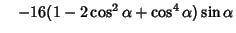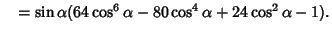(2)
Rewrite this using the identity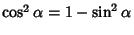,(3)
Now, letand, then(4)

which is a Cubic Equation in. The Roots are numerically found to be,,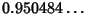. But, so these Roots correspond to,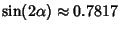,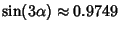. By Newton's Relation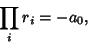(5)

we have(6)

or(7)

Similarly,(8)

The constants of the Cubic Equation are given by(9)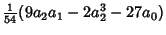(10)

The Discriminant is then(11)

so there are three distinct Real Roots. Finding the first one,(12)

Writing(13)

plugging in from above, and anticipating that the solution we have picked corresponds to,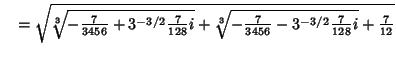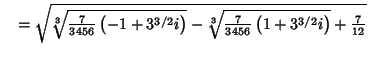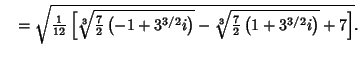(14)